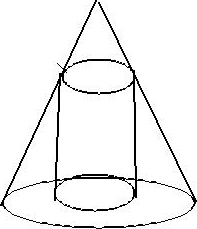# Optimization Problem

Calculus Level 4Let the maximum volume of a right circular cylinder that can be inscribed in a right circular cone of unit volume be $\dfrac ab$, where $a$ and $b$ are coprime positive integers. Find $a+b$.

×

Problem Loading...

Note Loading...

Set Loading...